# AQA A Level Maths: Pure复习笔记5.5.4 Inverse Trig Functions

### Inverse Trig Functions

#### What are arcsin, arccos and arctan?

• These functions are the inverse functions of sin, cos and tan
• sin (arcsin x) = x
• cos (arccos x) = x
• tan (arctan x) = x
• The domains of sin, cos, and tan must first be restricted to make them one-to-one functions (only one-to-one functions have inverses)

#### What are the restricted domains?

• domain of sin x is restricted to -π/2 ≤ x ≤ π/2  (-90° ≤ x ≤ 90°)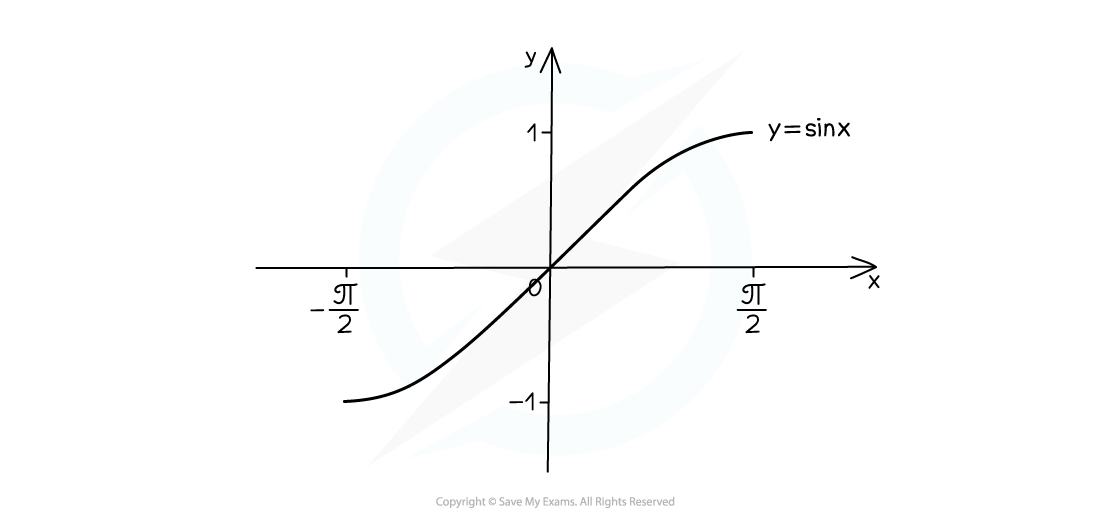• domain of cos x is restricted to 0 ≤ x ≤ π  (0° ≤ x ≤ 180°)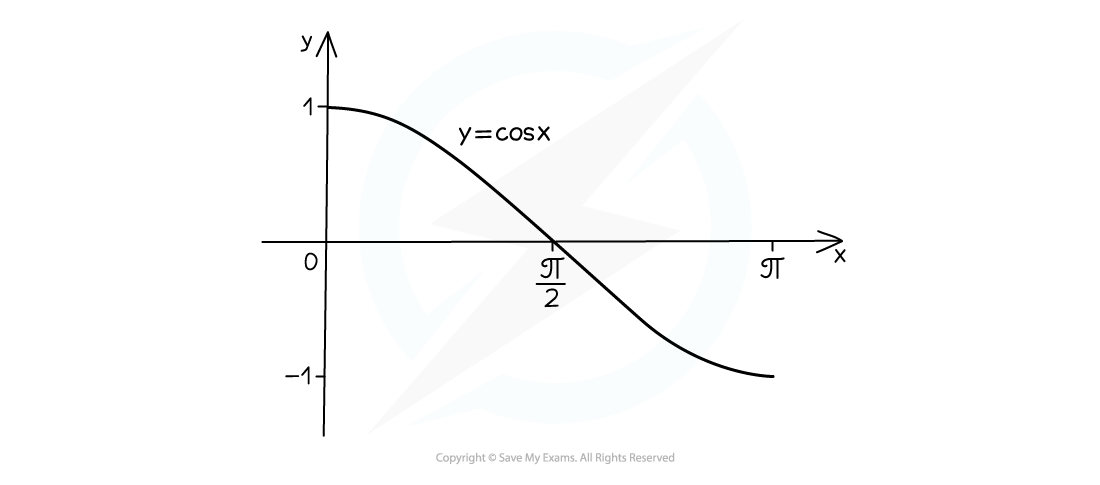• domain of tan x is restricted to -π/2 < x < π/2  (-90° < x < 90°)#### What does the graph of arcsin look like?

• The graph of y = arcsin x looks like this: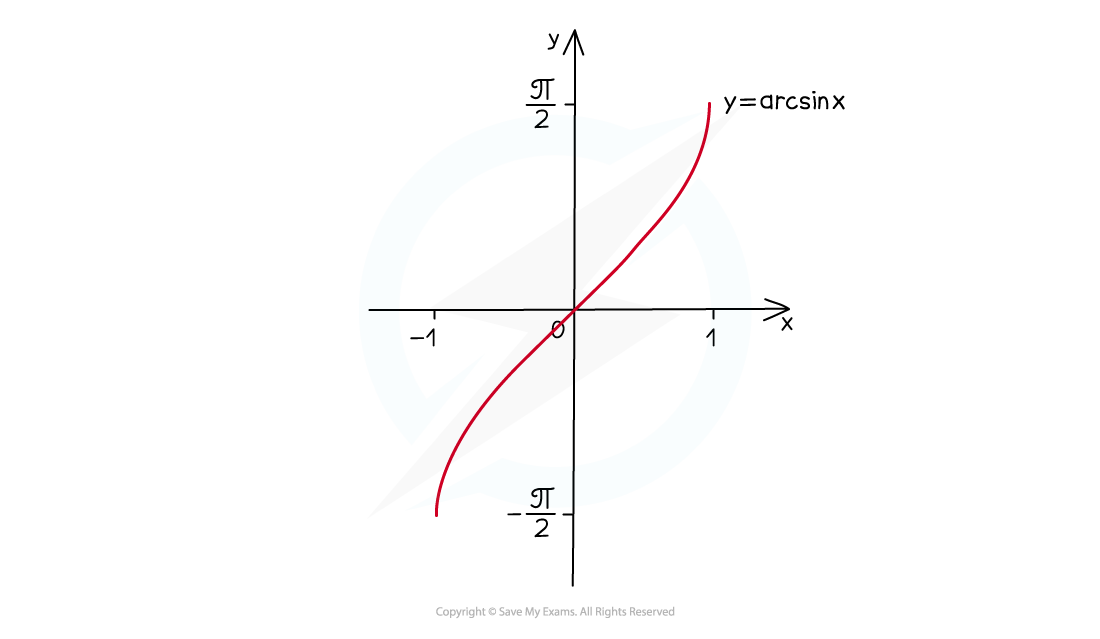• the domain is -1 ≤ x ≤ 1
• the range is -π/2 ≤ arcsin x≤ π/2  (-90° ≤ arcsin x ≤ 90°)

#### What does the graph of arccos look like?

• The graph of y = arccos x looks like this: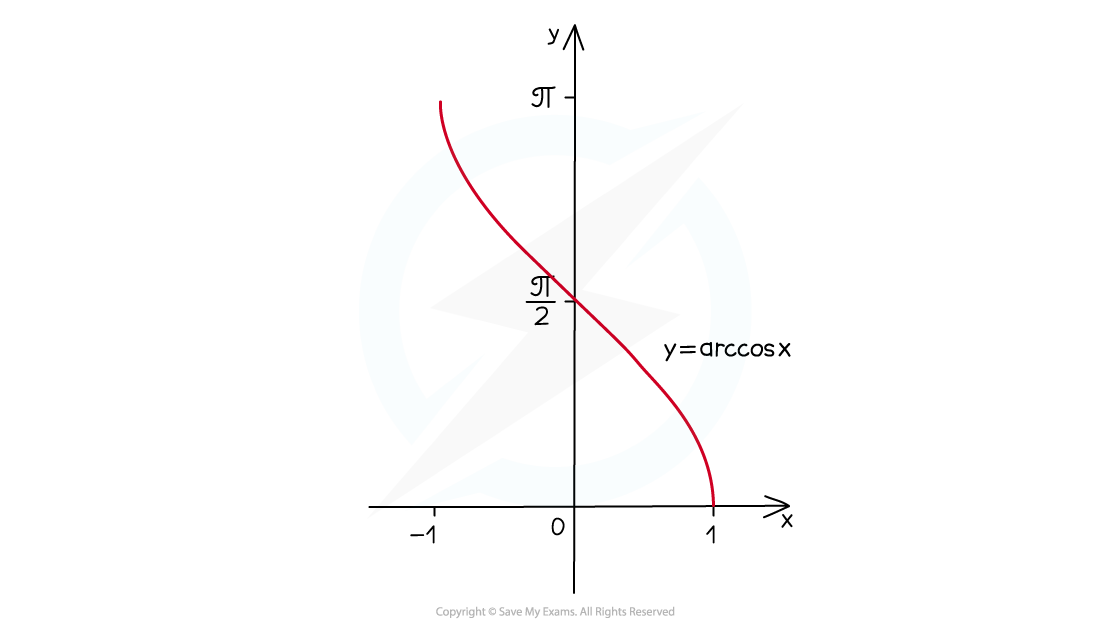•  the domain is -1 ≤ x ≤ 1
• the range is 0 ≤ arccos x ≤ π  (0° ≤ arccos x ≤ 180°)

#### What does the graph of arctan look like?

• The graph of y = arctan x looks like this: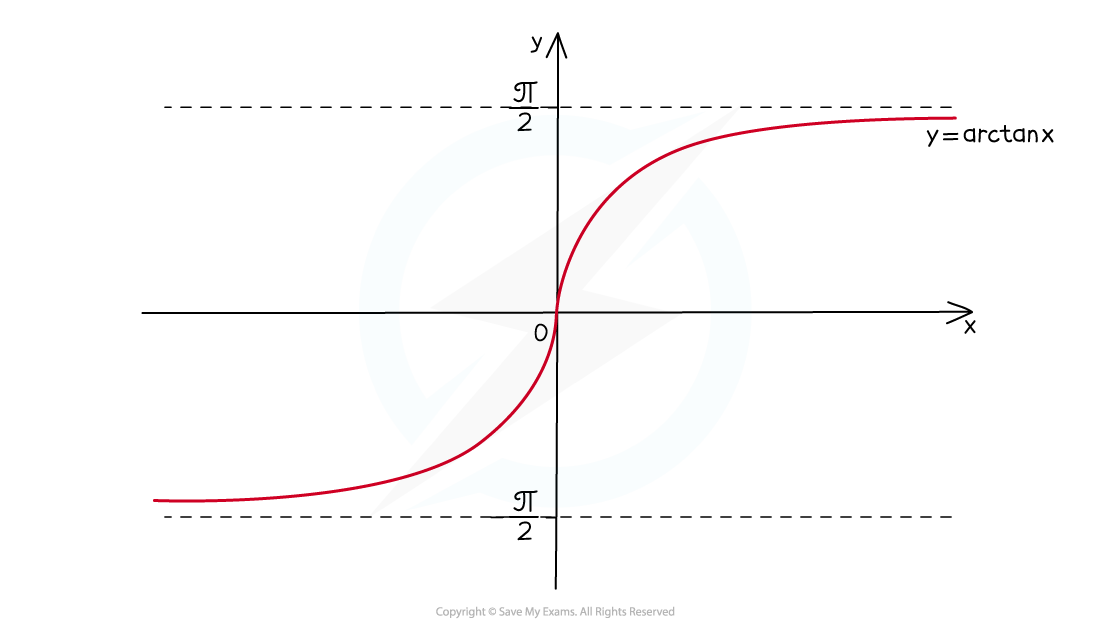• the domain is x ∈ ℝ  (ie arctan x is defined for all real number values of x)
• the range is -π/2 < arctan x < π/2  (-90° < arctan x < 90°)
• horizontal asymptotes at y= - π/2 and y = -π/2

#### Exam Tip

• Make sure you know the shapes of the graphs for sin, cos and tan.
• As inverses, the graphs of arcsin, arccos and arctan are reflections of sin, cos and tan in the line y = x.
• The values returned by the sin-1, cos-1 and tan-1 keys on your calculator are the values from the ranges of arcsin, arccos and arctan.

#### Worked Example